Request a Tool

Arcsin Calculator

Degree (°)
0

Formula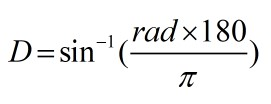• π = a constant value 3.1415
• D = Arcsin in degree

Defination / Uses

The inverse of the sine function is the arcsin function. It returns the angle whose sine is equal to a certain value. This means that the angle with a sin of 0.5 is 30 degrees. When you know the sine of an angle but not the actual angle, use arcsin.

0

Formula• M = Arcsin in milliradian

Defination / Uses

The inverse of the sine function is the arcsin function. It returns the angle whose sine is equal to a certain value. This means that the angle with a sin of 0.5 is 30 degrees. When you know the sine of an angle but not the actual angle, use arcsin.

0

Formula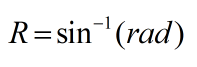• R = Arcsin in radian

Defination / Uses

The inverse of the sine function, whose value for a given argument between –1 and 1 is the angle in radians (between –/2 and –/2), and whose sine is that argument.

0

Formula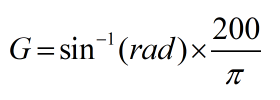• π = a constant value 3.1415
• G = Arcsin in Gradient

Defination / Uses

The inverse of the sine function is the arcsin function. It returns the angle whose sine is equal to a certain value. This means that the angle with a sin of 0.5 is 30 degrees. When you know the sine of an angle but not the actual angle, use arcsin.

Arcseconds
0

Formula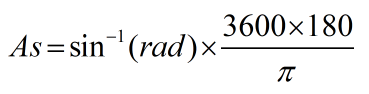• π = a constant value 3.1415
• As = Arcsin in Arcsecond

Defination / Uses

The angle arcsin x has the number x as its sine. Arcsin x is the arc whose sine is equal to x. Because the radian measure in the unit circle is the length of that arc... This is the principal values of y = arcsin x.

Arcminutes
0

Formula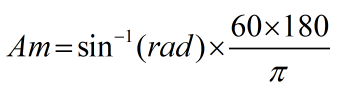• π = a constant value 3.1415
• Am = Arcsin in Arcminutes

Defination / Uses

The inverse of the sine function, whose value for a given argument between –1 and 1 is the angle in radians (between –/2 and –/2), and whose sine is that argument.

Circles
0

Formula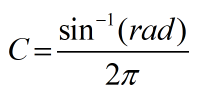• π = a constant value 3.1415
• C = Arcsin in Circles